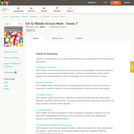Updating search results...

# 4 Results

View
Selected filters:Conditional Remix & Share Permitted
CC BY-NC-SA
Rating
0.0 stars

CK-12 Middle School Math Concepts for seventh grade provides a complete textbook. It presents topics including algebraic thinking, patterns, decimals, decimal operations, fractions, fraction operations, integers, integer operations, ratios, rates, proportions, percents, percent applications, equations, solving equations, inequalities, functions, graphing functions, geometry, plane geometry, solid geometry, area, perimeter, surface area, volume, statistics including mean, median, mode and range, graphing and types of graphs, and probability.

Subject:
Algebra
Geometry
Mathematics
Numbers and Operations
Statistics and Probability
Material Type:
Textbook
Provider:
CK-12 Foundation
Provider Set:
CK-12 FlexBook
Author:
Jen Kershaw
11/30/2012Conditional Remix & Share Permitted
CC BY-NC-SA
Rating
0.0 stars

Subject:
Mathematics
Material Type:
Activity/Lab
Author:
Jen Kershaw
Kimberly Hopkins
M Ed
03/24/2021Conditional Remix & Share Permitted
CC BY-NC-SA
Rating
0.0 stars

CK-12 Foundation's Middle School Math  Grade 6 Flexbook covers the fundamentals of fractions, decimals, and geometry. Also explored are units of measurement, graphing concepts, and strategies for utilizing the book's content in practical situations.

Subject:
Functions
Geometry
Mathematics
Material Type:
Textbook
Provider:
CK-12 Foundation
Provider Set:
CK-12 FlexBook
Author:
Brianne
Brockett
Colleen
Dan
Ggreenberg
Green
Jen
Kershaw
Mergerdichian
O'Donnell
Randy
Sarah
06/25/2011Conditional Remix & Share Permitted
CC BY-NC-SA
Rating
0.0 stars

A work in progress, CK-12's Math 7 explores foundational math concepts that will prepare students for Algebra and more advanced subjects. Material includes decimals, fractions, exponents, integers, percents, inequalities, and some basic geometry.

Subject:
Algebra
Functions
Geometry
Mathematics
Material Type:
Textbook
Provider:
CK-12 Foundation
Provider Set:
CK-12 FlexBook
Author:
Kershaw, Jen